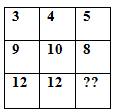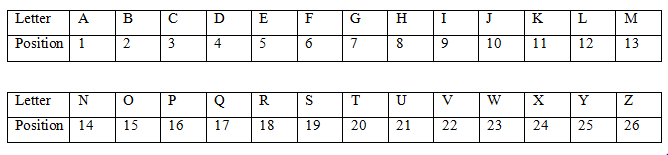# SSC CGL 2018 Practice Test Papers | Reasoning Ability (Day-30)

Dear Aspirants, Here we have given the Important SSC CGL Exam 2018 Practice Test Papers. Candidates those who are preparing for SSC CGL 2018 can practice these questions to get more confidence to Crack SSC CGL 2018 Examination.

[WpProQuiz 3106]

Click “Start Quiz” to attend these Questions and view Explanation

1)  In the English alphabet series if all vowels were dropped then which of the following letter will be the 8th to the right of 8th from the left end?

a) S

b) J

c) T

d) R

2) Select the related word/letters/number from the given alternatives.

26 : 16 :: 81 : ??

a) 20

b) 71

c) 18

d) 25

3)  In a certain code language “see eye jug” is coded as “ ju mu ra” and “eye in  ball  ” is coded as “ra la gi”, “ jug was long” is coded as “ju wa ba” then how long is coded?

a) wa

b) ba

c) Either a or b

d) ra

4) In a certain code language “see eye jug” is coded as “ ju mu ra” and “eye in  ball  ” is coded as “ra la gi”, “ jug was  long” is coded as “ju wa ba” then which of the following word is coded as eye?

a) ra

b) ju

c) mu

d) la

5)  In a certain code language “see eye jug” is coded as “ ju mu ra” and “eye in  ball  ” is coded as “ra la gi”, “ jug was long” is coded as “ju wa ba” then how “eye jug” is coded?

a) ra la

b) jura

c) mu la

d) waju

(6-8)) Five girls P, Q, R, S ad T sit in a straight line facing north direction. P sits to the immediate left of R. The number of persons between R and S is 2. Q has only one neighbor and the number of persons between Q and T is one.

6) Who sits in the middle of the line?

a) P

b) R

c) T

d) Q

7) Which of the following describes the position of T with respect to R?

a) Second to the left

b) Second to the right

c) Third to the right

d) Immediate left

8) In a certain way, some of the pairs belong to a category. Find the one which does not belong to that category.

a) (Q,S)

b) (S,T)

c) (P, R)

d) (S, P)

9)  Find the missing letter in the given table.a) 5

b) 9

c) 11

d) 3

10) Arranged the following words as per Dictionary order

(i) Starch

(ii) Stunt

(iii) Stock

(iv) stark

a) (iii), (ii), (iv), (i)

b) (iv), (iii), (ii), (i)

c) (iv), (i), (iii), (ii)

d) (i), (iv) ,(iii), (ii)(2+6) x 2=16

(8+1) x 2=18

Eye-ra

Jug-ju

See- mu

In-either la or gi

Ball- either la or gi

Was- either wa or ba

Long- either wa or ba

Eye-ra

Jug-ju

See- mu

In-either la or gi

Ball- either la or gi

Was- either wa or ba

Long- either wa or ba

Eye-ra

Jug-ju

See- mu

In-either la or gi

Ball- either la or gi

Was- either wa or ba

Long- either wa or ba

Arrangement is QSTPR

Arrangement is QSTPR

Except d all other pairs are neighbors and hence form a group.

3+9+12= 24

4+10+12=24

5+8+11=24

(i) Starch

(iv) stark

(iii) Stock

(ii) Stunt